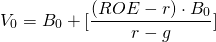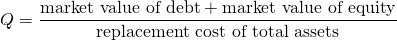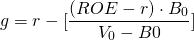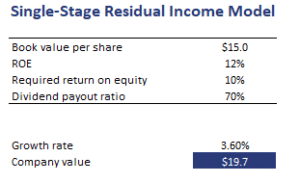## General Residual Income Model

The General Residual Income Model is a valuation model that does not make any assumptions regarding the long-term future earnings or dividend growth. Instead, the model makes the simplifying assumption that the company will pay a constant dividend and that the earnings growth will be constant. In that case, we can easily value the company by combining the current book value with the present value of residual income.

On this page, we discuss the residual income model formula, discuss the fundamental drivers of residual income, and finally provide a residual income model Excel calculator at the bottom of the page.

## Residual income model definition

The single stage residual income valuation model is defined as followswhere ROE is the return on equity, r is the required rate on return, B0 is the current book value per share, and g is the constant growth rate. It is evident that the model is closely related to the Gordon growth model

In the above formulation, the first terms is the current value of the company, which is assets minus liabilities. The second term is the present value of the expected future residual income.

## Residual income model fundamental drivers

The fundamental drivers of value under the residual income model are the following:

• If ROE equals the required rate of return on equity, the justified market value of the company is simply the book value. If the ROE is higher than the required rate of return, the company’s value will exceed the current book value
• The second term is the value added by the company because it is able to produce returns in excess of the required rate of return.

A closely related concept is the Tobin’s Q measure, which equalsAn important residual income model valuation disadvantage is the fact that growth is assumed to be constant indefinitely. In practice, however, residual income is expected to decrease because of increased competition. That is why the model is typically modified by forecasting the continuing residual income. An advantage of the approach is that we can easily modify the formula to calculate the implied growth rate in residual income.## General Residual Income model example

Let’s conclude with a short numerical example. The following table calculate a company’s value using a general residual income model Excel spreadsheet.## Summary

We discussed the single stage residual income valuation model. While the model is easy to use, analysts typically use a modified model that uses continuing residual income.